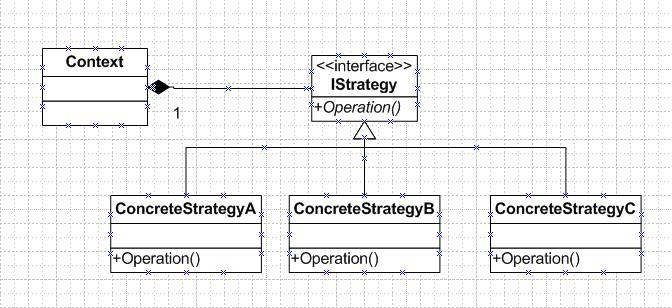面对现实，超越自己 逆水行舟，不进则退

Strategy（策略）模式又称Policy模式，用于定义一系列的算法，把它们一个个封装起来，并且使它们可相互替换。这里的算法并非狭义的数据结构或算法理论中所讨论的KMP、shell sort等算法，而是指应用程序设计中不同的处理逻辑，前面所说的狭义的算法只是其中的一部分。Strategy模式使得算法与算法的使用者相分离，减少了二者间的耦合度，使得算法可独立于使用它的客户而变化；同时，由于设计粒度的减小，程序的复用性也得到了进一步提高，分离出来的算法可以更好地适应复用的需要。

Strategy模式的结构如下图所示：State模式在于将其状态信息分离出来保存到一个独立的对象中，以便状态信息的获取或状态的转换；Strategy模式在于将可能的算法分离出来，根据需要进行适当的选择。此外，二者的区别还在于，Strategy模式中各个Strategy（算法、策略）往往用于解决相同的问题，即只是解决同一问题的不同“策略”、“途径”，而且，一次只能有一个Strategy为上次应用提供服务；而State模式中的各个State本身往往具有一定的差异，但他们之间存在明显的相互转换的关系，而且这种转换往往会在程序运行过程中经常性地发生，同时存在一个以上State也是可能的。

1.

2.

3.

4.

1.

2.

3.

1 #include <iostream>
2 #include <vector>
3 #include <algorithm>
4 #include <time.h>
5 using namespace std;
6
7 template <typename T>
8 class SortStrategy    // Strategy
9 {
10 public:
11     virtual void Sort( vector<T>& v_t ) = 0;
12 };
13
14 template <typename T>
15 class SortQuick : public SortStrategy<T>    // ConcreateStrategy1
16 {
17 public:
18     void Sort( vector<T>& v_t ) { std::sort( v_t.begin(), v_t.end() ); }
19 };
20
21 template <typename T>
22 class SortStable : public SortStrategy<T>    // ConcreateStrategy1
23 {
24 public:
25     void Sort( vector<T>& v_t ) { std::stable_sort(v_t.begin(), v_t.end()); }
26 };
27
28 template <typename T>
29 class Context {        // Context, who or whose client takes charge of which strategy will be selected
30 public:
31     Context() { m_pStrategy = NULL; }
32     virtual ~Context() { if (m_pStrategy != NULL) delete m_pStrategy; }
33
34     void SetStrategy(SortStrategy<T>* pStrategy);    // select a strategy
35
37     bool SortVector();
38     void OutputVector();
39 private:
40     vector<T> m_vt;
41     SortStrategy<T>*  m_pStrategy;    // a pointer to current strategy
42 };
43
44 template <typename T>
45 void Context<T>::SetStrategy( SortStrategy<T>* pStrategy )
46 {
47     if ( NULL != m_pStrategy )
48         delete m_pStrategy;
49
50     m_pStrategy = pStrategy;
51 }
52
53 template <typename T>
55 {
56     m_vt.clear();
57     copy( v_t.begin(), v_t.end(), back_inserter( m_vt ) );
58 }
59
60 template <typename T>
61 bool Context<T>::SortVector()
62 {
63     if ( NULL == m_pStrategy )
64         return false;
65
66     m_pStrategy->Sort( m_vt );
67
68     return true;
69 }
70
71 template <typename T>
72 void Context<T>::OutputVector()
73 {
74     copy( m_vt.begin(), m_vt.end(), ostream_iterator<T>( cout, " " ) );
75 }
76
77 // a functor to generate random int
78 struct RandGen
79 {
80     RandGen(int ratio) { m_ratio = ratio; }
81     int operator() () { return rand() % m_ratio + 1; }
82 private:
83     int m_ratio;
84 };
85
86 int main()
87 {
88     const int NUM = 9;
89     vector< int > vi;
90     time_t t;
91     srand( (unsigned) time(&t) );
92
93     // create a vector with random information
94     vi.reserve(NUM + 1);
95     generate_n(back_inserter(vi), NUM, RandGen(NUM));
96
97     Context< int >  con;
98     con.SetStrategy( new SortQuick<int>() );
100     con.OutputVector();
101
102     cout << endl;
103
104     con.SortVector();
105     con.OutputVector();
106
107     return 0;
108 }

posted on 2012-07-17 08:11 王海光 阅读(399) 评论(0)  编辑 收藏 引用 所属分类: Design Pattern